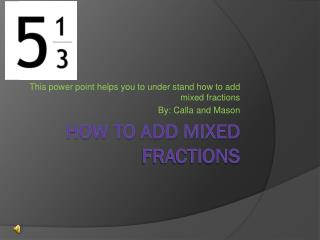DownloadDownload Presentation# How To Add Mixed Fractions

Download Presentation## How To Add Mixed Fractions

- - - - - - - - - - - - - - - - - - - - - - - - - - - E N D - - - - - - - - - - - - - - - - - - - - - - - - - - -
##### Presentation Transcript

1. This power point helps you to under stand how to add mixed fractions By: Calla and Mason How To Add Mixed Fractions

2. 1. When you add a mixed fraction to make it easier you should write the problem. Lets say the fraction you are working with is 6 2/7 + 5 3/14 =?

3. 2. You want to write it step by step such as… 6 + 5 + 2/7 + 3/14

4. 3. Now that we wrote our problem out you want to add the whole numbers 6 + 5 = 1111 is the answer of 6 + 5

5. 4. Now you need to add the fractions 2/7 + 3/14 = ?The denominators are not the same so now we need to find a lcd{lowest Common Denominator}

6. 5. Now we are going to find our LCD 7: 7 , 14 , 21 , 28 , 3514: 14Now that we found a LCD we can multiply to get the fractions we need to add

7. 6. Now we need to multiply to get the fractions we need Now we need to get 2/7 to a denominator of 14 we do not Need to DO THIS with 3/14 because that already has a denominator of 14.2/7 seven goes into 14 two times. Now that we know how many times Seven goes into 14. Since 7 goes into 14 two times now we multiply the numerator by how many times the Denominator goes into the LCD. 2 x 2= 4 now we add 4/14 + 3/14= ?

8. 7. Add the fractions 4/14 + 3/14 = 7/14 now we need to simplify.½ is simplified because 7 is half of 14

9. 8. Add whole numbers and fractions Our Answer is 11 ½ and not 12 ½ because our fraction answer is not 1 ½

10. 9. Answer 11 + ½ = 11 ½

11. Hope you understand

12. That is our tutorial on how to add mixed fractions!!!!!!!!!!!!!!!!!!!!!!!!!!!!